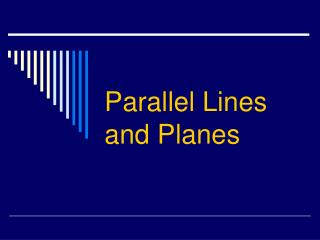DownloadDownload PresentationParallel Lines and Planes

# Parallel Lines and Planes

Télécharger la présentation## Parallel Lines and Planes

- - - - - - - - - - - - - - - - - - - - - - - - - - - E N D - - - - - - - - - - - - - - - - - - - - - - - - - - -
##### Presentation Transcript

1. Parallel Lines and Planes

2. Section 3 - 1 Definitions

3. Parallel Lines - coplanar lines that do not intersect

4. Skew Lines - noncoplanar lines that do not intersect

5. Parallel Planes - Parallel planes do not intersect

6. THEOREM 3-1 If two parallel planes are cut by a third plane, then the lines of intersection are parallel.

7. Transversal - is a line that intersects each of two other coplanar lines in different points to produce interior and exterior angles

8. ALTERNATE INTERIOR ANGLES - two nonadjacent interior angles on opposite sides of a transversal

9. Alternate Interior Angles 4 2 3 1

10. ALTERNATE EXTERIOR ANGLES - two nonadjacent exterior angles on opposite sides of the transversal

11. Alternate Exterior Angles 8 6 7 5

12. Same-Side Interior Angles - two interior angles on the same side of the transversal

13. Same-Side Interior Angles 4 2 3 1

14. Corresponding Angles - two angles in corresponding positions relative to two lines cut by a transversal

15. Corresponding Angles 4 8 2 6 7 3 1 5

16. 3 - 2 Properties of Parallel Lines

17. Postulate 10 If two parallel lines are cut by a transversal, then corresponding angles are congruent.

18. THEOREM 3-2 If two parallel lines are cut by a transversal, then alternate interior angles are congruent.

19. THEOREM 3-3 If two parallel lines are cut by a transversal, then same-side interior angles are supplementary.

20. THEOREM 3-4 If a transversal is perpendicular to one of two parallel lines, then it is perpendicular to the other one also.

21. Section 3 - 3 Proving Lines Parallel

22. Postulate 11 If two lines are cut by a transversal and corresponding angles are congruent, then the lines are parallel

23. THEOREM 3-5 If two lines are cut by a transversal and alternate interior angles are congruent, then the lines are parallel.

24. THEOREM 3-6 If two lines are cut by a transversal and same-side interior angles are supplementary, then the lines are parallel.

25. THEOREM 3-7 In a plane two lines perpendicular to the same line are parallel.

26. THEOREM 3-8 Through a point outside a line, there is exactly one line parallel to the given line.

27. THEOREM 3-9 Through a point outside a line, there is exactly one line perpendicular to the given line.

28. THEOREM 3-10 Two lines parallel to a third line are parallel to each other.

29. Ways to Prove Two Lines Parallel • Show that a pair of corresponding angles are congruent. • Show that a pair of alternate interior angles are congruent • Show that a pair of same-side interior angles are supplementary. • In a plane show that both lines are  to a third line. • Show that both lines are  to a third line

30. Section 3 - 4 Angles of a Triangle

31. Triangle – is a figure formed by the segments that join three noncollinear points

32. Scalene triangle – is a triangle with all three sides of different length.

33. Isosceles Triangle –is a triangle with at least two legs of equal length and a third side called the base

34. Angles at the base are called base angles and the third angle is the vertex angle

35. Right triangle – is a triangle with one right angle (90°)

36. Auxillary line – is a line (ray or segment) added to a diagram to help in a proof.

37. THEOREM 3-11 • The sum of the measures of the angles of a triangle is 180

38. Corollary • A statement that can easily be proved by applying a theorem

39. Corollary 1 • If two angles of one triangle are congruent to two angles of another triangle, then the third angles are congruent.

40. Corollary 2 • Each angle of an equiangular triangle has measure 60°.

41. Corollary 3 • In a triangle, there can be at most one right angle or obtuse angle.

42. Corollary 4 • The acute angles of a right triangle are complementary.

43. THEOREM 3-12 • The measure of an exterior angle of a triangle equals the sum of the measures of the two remote interior angles.

44. Section 3 - 5 Angles of a Polygon

45. Polygon – is a closed plane figure that is formed by joining three or more coplanar segments at their endpoints, and

46. Each segment of the polygon is called a side, and the point where two sides meet is called a vertex, and